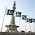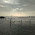## Saturday, 12 May 2012

### Factor and reminder theorem :Polynomial class IX

Proof of this factor theorem
Let p(x) be a polynomial of degree greater than or equal to one and a be areal number such that p(a) = 0. Then, we have to show that (x – a) is a factor of p(x).
Let q(x) be the quotient when p(x) is divided by (x – a).
By remainder theorem
Dividend = Divisor x Quotient + Remainder
p(x) = (x – a) x q(x) + p(a) [Remainder theorem]
p(x) = (x – a) x q(x) [p(a) = 0]
(x – a) is a factor of p(x)
Conversely, let (x – a) be a factor of p(x). Then we have to prove that p(a) = 0
Now,     (x – a) is a factor of p(x)
p(x), when divided by (x – a) gives remainder zero. But, by the remainder theorem, p(x) when divided by (x – a) gives the remainder equal to p(a). p(a) = 0
Proof of remainder theorem.
Let q(x) be the quotient and r(x) be the remainder obtained when the polynomial p(x) is divided by (xa).
Then, p(x) = (xaq(x) + r(x), where r(x) = 0 or some constant.
Let r(x) = c, where c is some constant. Then
p(x) = (xaq(x) + c
Putting x = a in p(x) = (xaq(x) + c, we get
p(a) = (a–a) q(a) + c
p(a) = 0 x q(a) + c p(a) = c
This shows that the remainder is p(a) when p(x) is divided by (xa).
1. Factories
(i)   a2-b2-4ac+4c2                              (ii) 7x2 + 2 √14x + 2
(iii) 4a2-4b2+4a+1                                (iv)  x4+y4-x2y2
(v) x- x3                                                                       (vi)  x3-5x2-x+5
(vii)  x2+3√3x +6                                 (viii)  a3(b-c)3+b3(c-a)3+c3(a-b)3
(ix)  .8a3-b3 -12a2 b +6ab2                            (x) b.4x2 +9y 2+ 25z2 -12xy - 30yz +20xz
(xi)  x 3 + 4x 2 + x – 6                         (xii)  4x4 + 7x2 – 2
(xii)  x 2 - 2√3x – 45                             (xiii)  3 - 12(a - b)2
Q. Find degree of 5x3 -6x3y+10y2+11                        
Q. find the value of k if(x-2) is a factor of p(x)=k x2 -- √2x +1                       [ (2√2- 1)/4 ]
Q. Find the remainder when x3+3x2+3x+1 when divided by 3x+1.
Q.  if x-1 and x-3 are the factors of p(x) x (raise to the power 3)-a x (raise to the power 2)-13x-b then find the value of a and b
Q. If(X2-1) is a factor of ax4+bx3+cx2+dx+e,show that  a + c + e = b + d =0
Q. prove that (x+y)3-(x-y)3-6y(x2-y2)=8y3
Ans:  x+ 3x2y + 3xy+ y3 - (x- 3x 2y + 3xy- y3) - 6yx+ 6y3           [(a + b)3 = a3 + 3a2b + 3ab2 + b3
(a - b)3 = a3 - 3a2b + 3 ab2 - b3]  = x+ 3x2y + 3xy+ y3 - x+ 3x2y - 3xy+ y- 6yx+ 6y3       = 2y+ 6 y3        = 8y3
Q. The polynomials {ax3-3x2+4} and {3x2-5x+a} when divided by {x-2} leave remainder "p" and "q" respectively. if p-2q+=a find the values of "a".                          [a is –8.]
Q. If ( x − 4) is a factor of the polynomial 2 x 2 + Ax + 12 and ( x − 5) is a factor of the polynomial x 3 − 7 x 2 + 11 x + B , then what is the value of ( A − 2 B )?
Q. f x -1/x =3; then find the value of x3 -1/x3                                                       
Q. if a+b+c=7 and ab+bc+ca=20 find the value of (a+b+c)2
Q. The polynomial f(x)=x4-2x3+3x2-ax+b when divided by (x-1) and (x+1) leaves the remainders 5 and 19 respectively. Find the values of a and b. Hence, find the remainder when f(x) is divided by (x-2)
Q. check:  2x +1 is a factor of p(x)=4x3 + 4x2 - x -1

1.2.Ummm... awwesome practice papers but...there is a scope of improvement

3.super.....................................................................................................................

4.5.